# How to interface a 3.3V output to a 5V input.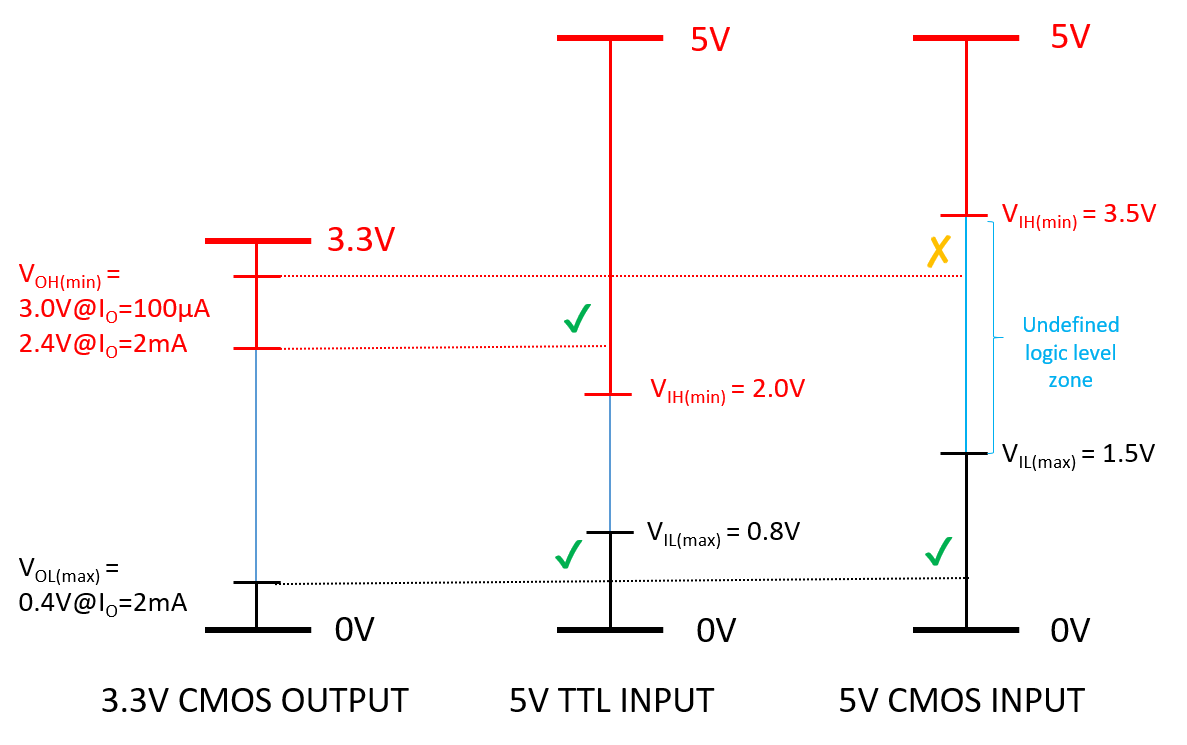### Introduction

In a previous article we dealt with the problem of interfacing a 5V output signal to a 3.3V system. In this article we cover the opposite problem: we have a 3.3V output and we need to drive a 5V system.

This is a very typical situation in which we have a 3.3V system (e.g. most of 32-bit systems, such as uChip), and we need to send data to an older 5V system.

First of all, we need to consider which kind of 5V system we are interfacing. In particular, we need to know:

1. The low and high level input and output voltages.
2. The input current.

For CMOS inputs, the input current is typically around 1uA or less, therefore there is no such a concern. For TTL devices, the input current might be even more than 1 mA (see for instance 7400 datasheet). Therefore, when interfacing with TTL inputs, some additional care should be taken, as we will explain on a case-by-case basis.

Another, and much more important, aspect is represented by the logic levels.

In fact, 5V TTL and 5V CMOS inputs have different logic levels, therefore some of the solutions we will present will be adequate for some inputs, but these could not work reliably for other inputs types.Fig.1 Logic levels of a 3.3-V CMOS output, a 5-V TTL input and a 5-V CMOS input.

The main ways to interface a 3.3V output to a 5V input are:

• Direct connection
• Using a 74HCTxx gate (or other 5-V TTL-input compatible families)
• Using a diode offset
• Resistor Offset
• BJT/MOSFET inverter
• Series MOSFET
• Series BJT
• Level Translator IC
• Optocoupler/Isolator

### DIRECT CONNECTION

This is the simplest way. This solution “almost always” works, but with some important warnings.

First, when interfacing with TTL inputs, any “modern” CMOS output will work, as the high level output voltage of a 3.3V CMOS is close to 3.3V (note! The actual output voltage depends on the output current. For heavily loaded outputs, the output levels might vary of 0.5V or more!), still the minimum high-level input voltage for a TTL is 2V. Similarly, if not too much heavily loaded, the low-level output voltage of a CMOS is lower than the maximum low-level TTL input voltages.

We wrote “modern” CMOS because older CMOS chips (e.g. CD4xxx series) have a very high output impedance, therefore they cannot sink/source too much current (you generally do not want to sink/source more than 0.5 mA). Trying to get too much current will make the output voltage shift too much. Older TTL chips have an input current, which might exceed 1mA. Almost all modern CMOS devices (e.g. the GPIOs of MCUs) allows driving a much higher current without problems.

Second, when interfacing to a 5V CMOS devices, this might work, but not reliably. In fact, the high level input voltage of a 5V CMOS is 3.5V. This is even higher than the maximum output voltage you might expect from a 3.3V system (i.e. 3.3V).
Still, why this generally works ? The answer is due to the actual threshold logic level, which is 2.5V for a 5V CMOS. Any voltage above 2.5V would be read as 1, and any voltage below 2.5V would be read as 0.
However, the actual threshold level might shift with temperature and aging: operating between the two logic levels region is not safe. Any noise or disturbance might produce a glitch at the output. If your system must work reliably, then you need other solutions, as shown below.

Furthermore care should be taken when driving a digital non hysteretic input close to the logic level threshold, as current consumption would occur. In fact, consider the simple CMOS inverter, shown below: when the input voltage is close to the VDD/2, both MOSFETs are in the ON state, therefore a direct path current will flow from VDD to GND.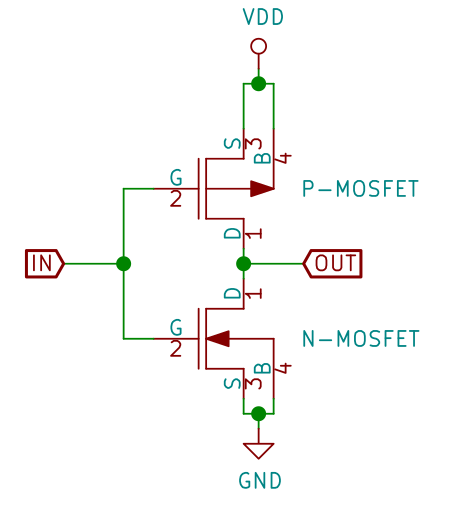Fig.3. The internal circuit of a CMOS inverter. If the voltage at the “IN” signal is close to VDD/2, then both MOSFET will be in the ON state, and current will flow between VDD and GND..

• Fast

• Reduced noise margins.
• Works reliably only with some logic families.

### USING A 74HCTxx GATE (or any other logic family with TTL compatible inputs)

The 74HCTxx family series are CMOS devices with TTL compatible logic levels (all the other 5V logic families that have TTL compatible input levels will work too) . In particular, the input high-voltage level is 2V, which is well below the CMOS high output voltage. By inserting any logic gate (see examples below) with TTL-compatible input levels between your systems, you create a suitable voltage level translator.

• Fast
• Works with both CMOS and TTL devices
• Need only one power supply.

• Requires an external IC (and possibly its decoupling capacitor)

### USING A DIODE OFFSET

With the direct connection to a 5V CMOS input, we saw that the main issue was the high level output voltage of the 3.3V output, which is not high enough to be just in the safe region (3.3V at most, vs 3.5V minimum). Instead, the maximum voltage of a low level CMOS input is 30% of VDD, i.e 1.5V in a 5V system. Therefore, if we could add small offset to the CMOS output, that would be great. For this reason, one could simply ad a diode and a pull-up resistor.

However, in this way, current will flow into the output protection diodes of our 3.3V system. Such current should be made as small as possible, to avoid damaging the 3.3V system.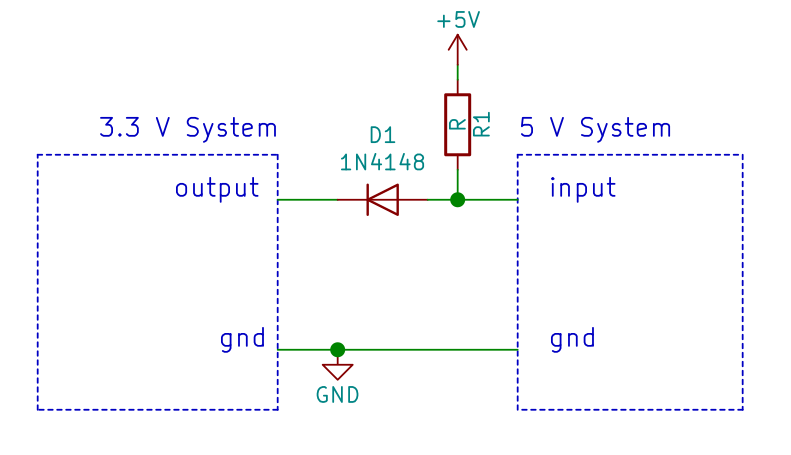Fig. 5. The circuit above introduces a 0.7 to 1V offset (depending on R1 value). However, reverse current will flow into the output even when it is in the high state. This might cause issues on the 3.3V device.

A better solution is to use an additional diode. Since the new diode is directly connected to the 3.3V rail (it does not have to pass through our IC), the current will flow preferably there.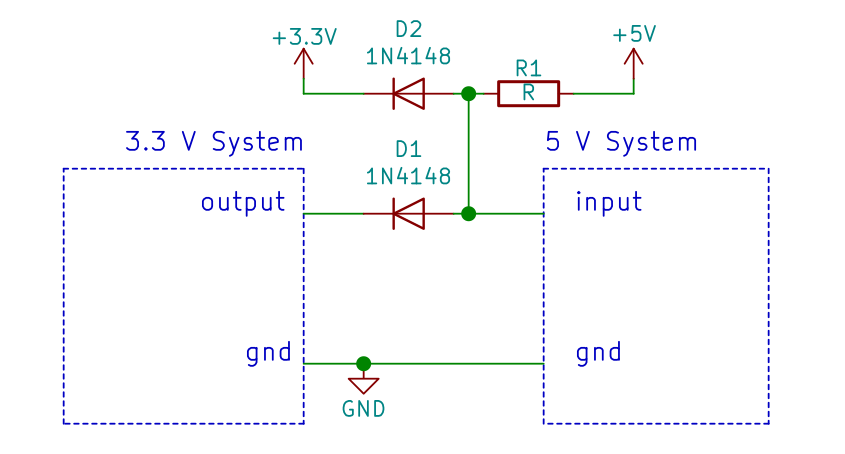Fig. 6. In this circuit, when the output is high, the current will not flow into the output, but across D2.

Still, both these solutions has an intrinsic problem: if the 3.3V system is really low power, then of course it will consume a very low current. If the total current consumption is lower than the current flowing into the resistor, then effectively, the 3.3V rail will be powered by the 5V, through the resistor and diode.

This might be an issue, as, if no enough current is drawn by the 3.3V system, the 3.3V voltage might increase up to about 4.3V, which could damage the 3.3V system itself.

A simple solution is to put a second resistor, which draws at least the current that would flow into D2 (about 1V/R1. Therefore R2 should be 3.3 times R1 or less).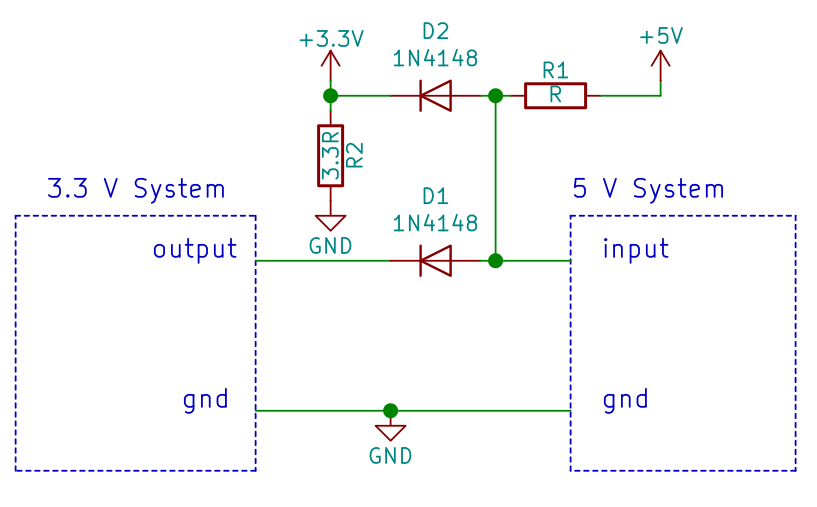Fig. 7. Adding R2, with a value at most 3.3 times larger than R1, we are sure that the current flowing into D2 will be “dissipated”, and will not increase the 3.3V voltage.

The value of the pull-up resistor should be calculated so that:

• It is low enough to grant us the desired speed.
• It is much smaller than the input impedance (though in a CMOS device, this is not much an issue).
• It is large enough, not to overload the CMOS output voltage, especially at low level. This is especially an issue on those CMOS output with relatively high output impedance (CD40xx series).
• It is large enough, to avoid too much current flowing into the 3.3V rail.
• It is large enough, to keep current consumption at an acceptable level.

• Cheap

• Much slower than other solutions.
• Requires a careful resistor value selection: to avoid damage, to get a decent speed, and to keep the high and low voltage within the correct ranges.
• Relatively high current consumption.
• Requires 2 to 4 additional components.
• Poor noise margins.
• Requires a low impedance driving output.
• Requires a relatively high input impedance

### RESISTOR OFFSET

We can introduce an offset also using a resistor divider too.

This simple solution is cheaper (but somewhat slower) than the diode-offset, and still has the problem of current flowing into the output pin.

A better solution is to add a dummy load to the output, that will adsorb the current coming from the 5V through R1 and R2. Another way to view this, is that, disconnecting the output, with the calculated value, R1-R2-R3 will form a resistor divider and the voltage across R3 would be 3.3V at most. The values indicated in the figures are expressed in terms or a generic “R” value.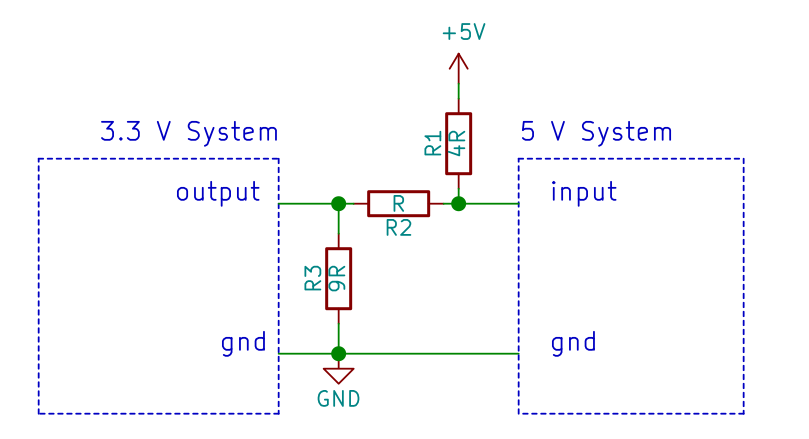Fig. 9. Adding R3 will allow to shunt to ground any current coming from the 5V (instead to 3.3V through the output pin), when the output is at 3.3V.

When the output is 0, the voltage will be 5V * (R2/(R1+R2)), i.e. 1V, which is below the 1.5V threshold. When the output is 3.3V, the voltage will be 5V * (R2/(R1+R2)) + 3.3V * (R1/(R1+R2)) = 3.64V. Better high-level values can be achieved  by adjusting the R1/R2 ratio, but you must take into account that the voltage should be smaller than 1.5V, when the output is at 0V.

Note: we took 0 and 3.3V as the CMOS output voltages, when the output is low and high, respectively. While, this time, there is no problem (unless R3 is too low) about the high level voltage (as it is pulled up by R1+R2), the low-level voltage will increase according to the current flowing into the output.

• Cheaper than diode offset.

• Slower than the diode offset solution, especially in the high-to-low transition, as the current flows across R1 and R2, which will have a much higher impedance, with respect to a diode.
• Requires careful resistor value selection, to avoid damage, to get a decent speed and to keep the high and low voltage within the correct ranges.
• Relatively high current consumption.
• Requires 2 to 3 additional components.
• Poor noise margins.
• Requires a low impedance driving output.
• Requires a relatively high input impedance.

### BJT/MOSFET INVERTER

As we did in the other article, a simple MOSFET/BJT can be used, if an inverted signal can be accepted or is desired. Otherwise, an additional stage can be used.

• Much simpler dimensioning, with respect to the diode offset.
• Better noise margin, as both the low and high levels are close to the rails.

• Requires 2/3 external components.
• It is inverting.
• Relatively slow low-to-high rise time.
• The BJT implementation is actually relatively slower, with respect to MOSFET implementation, due to the relatively slow BJT turn-off characteristics.
• Relatively high consumption, when the MOSFET/BJT is in the ON state.
• Requires a relatively high input impedance

### SERIES MOSFET

In the 5-to-3.3V article, we showed this little circuit, and we told that it is bidirectional. Indeed, it can be used for 3-to-5V translation too!

The working principle is simple. When the output is at 3.3V, the MOSFET will be in the OFF-state, as VGS=0V, therefore the output is held at 5V by the pull-up resistor. If the output is low, then VGS is 3.3V. Assuming a MOSFET with a logic-level threshold (it should be fully-on when VGS = 2.5V), the MOSFET will turn on, passing the low-level value to the 5V input.

• Bidirectional
• Relatively simple solution.
• It does not invert the input, as the single MOSFET/BJT in common source configuration.

• Requires 2 external components
• Relatively slow.
• Requires a low-impedance driving output to avoid overload.
• Relatively high power consumption.
• Requires a relatively high input impedance.

### SERIES BJT

This is the brother of the previous solution, except it uses a BJT. The working principle is the same (as we also explained in the previous article). It shares the same benefits of the previous circuit, but it also introduces some additional drawbacks.

• Bidirectional
• Relatively simple solution.
• It does not invert the input, as the single MOSFET/BJT in common source configuration.

• Requires 3 external components.
• Relatively slow.
• Requires a low-impedance driving output to avoid overload.
• Relatively high power consumption.
• Requires a relatively high input impedance.
• The BJT saturation collector-to emitter voltage (VCESAT) is added to the low-level output voltage. This is normally not a big deal, though.

### LEVEL TRANSLATOR IC

A dedicated level translator IC, such as 74LVC1T245, will do everything you need, with better performances with respect to discrete solutions, but at a much higher price.

There are many variants, such as with more channels (74LVC8T245, 74LVC16T245), or different logic family (74ALVT162245), with different speeds (and prices).

Use this solution when you require a high performance 3.3V to 5V level translation (typically in high speed buses, clocks, etc.)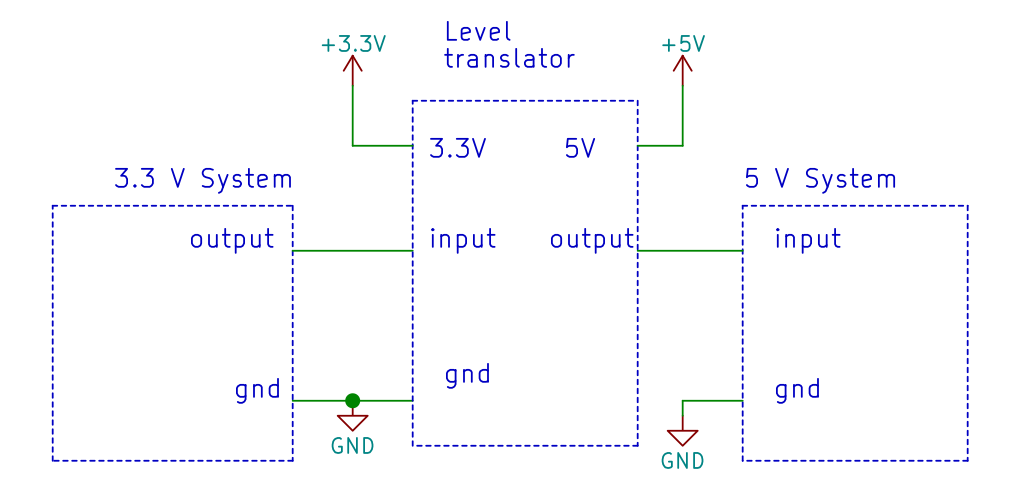Fig. 14. A level translator will generally perform better with respect to the other solutions, especially in terms of noise margin and speed (except direct connection).

• Fast (even though not as fast as the direct connection, as a small delay is added).
• High noise margin.

• Requires a level shifter and possibly 2 decoupling capacitors (one per power domain).
• Expensive.

### OPCOUPLER/ISOLATOR

As shown in the previous article, this solution is “any voltage-to any voltage” translator, therefore it can be used also for the 3.3V to 5V translation. There are 4 configurations, depending on your requirements.

Beware that some configurations require a strong low-level output driver (while nothing is requested in terms of high-level output strength), while the other requires a strong high-level output driver.

Similarly, the output will provide a strong pull-up/down path (through the coupler), and a weaker one (through the pulldown/up resistor, respectively).

Instead of using standards optoisolators, you can use more recent devices, based on capacitive, giant magnetoresistive or magnetic couplings, even though these are much more expensive, typically.

• Electrical insulation.
• Better safety.
• “Any voltage to any voltage” conversion.
• You can optionally invert the signal.

• Typically slow, except when high speed isolators are used.
• Relatively expensive.
• Quite bulky device.
• High power consumption.
• You have some constraints on the output and input impedances.

## Conclusions

We have shown some of the main methods of interfacing a 3.3V to a 5V system! We will keep updating this post if we find some other interesting techniques! For the opposite direction, i.e. 5V to 3.3V interfacing, refer to this article!

1.Jarkko Sonninen

Nice article.
I think there is a copy-paste error in Fig 4. 5V and 3.3V should be swapped.

1.N W (Post author)

Thank you very much, the figure has now been corrected!

2.Paul B.

Figure 15b and 16a are wrong – show 3.3 V on the right hand side!

1.next-hack (Post author)

Thank you Paul, we have corrected them right now!

3.Tom

For Figure 10 – there is a way to have a non-inverting configuration. If you connect pin 2 to +3.3V, and pin 3 to your 3.3v signal. With appropriate resistors, you get a non-inverted signal.

1.next-hack (Post author)

Hi, that’s shown in Fig 13 🙂

This site uses Akismet to reduce spam. Learn how your comment data is processed.